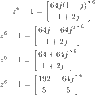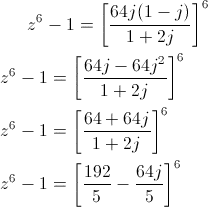# The distinct roots of complex number

• timeforchg

#### timeforchg

I am trying to find the z0 to z6 roots of this equation but I am stuck here. Anyone care to show the step by step on how to procced?

#### Attachments

•gif.latex.gif
2.9 KB · Views: 657

Welcome to PF;

is it that z=x+iy: z6-1=c6 (c=a+ib): a,b,x,y are real, a,b are constants?
so you need to find:

$(x+iy)^6-(a+ib)^6-1=0$

You could multiply out the polynomials and then solve for the real and imaginary parts ... or express each of the complex numbers as exponentials. Which have you tried?

I am trying to find the z0 to z6 roots of this equation but I am stuck here. Anyone care to show the step by step on how to proceed?

## The Attempt at a SolutionIt can be easier to take the power of a complex number when it's expressed in "polar" form.

Assuming your last line is correct:

$\displaystyle \frac{192}{5}-j\frac{64}{5}=\frac{64\sqrt{10}}{5}e^{-j\,\tan^{-1}(1/3)}$

Hi SammyS,

How do you derive to that equation?

Hi SammyS,

How do you derive to that equation?
That's polar form for a complex number. (By the Way, I'm not too sure it will be all that helpful here, what with the -1 part of the z6 - 1, and with the arctan in the exponent.)

In general, you can express the complex number, a + b j as
$a+bj=\sqrt{a^2+b^2\ }\left(\cos(\theta)+j\sin(\theta)\, \right)\,,$
where $\displaystyle \tan(\theta)=\frac{b}{a}\ .$

Using Taylor series, it can be shown that $\displaystyle e^{j\,\theta}=\cos(\theta)+j\sin(\theta)\ .$

$\displaystyle \cos(\theta)+j\sin(\theta)$ is also called the cis() function, but the exponential form makes it very handy for taking a complex number to a power.
$\displaystyle \frac{192}{5}-j\frac{64}{5}=\frac{64}{5}\left(3-1j\right)$

$\displaystyle 3-j=\sqrt{3^2+1^2}\left(\cos(\theta)+j\sin(\theta) \right)\,,\ \text{ where }\theta=\arctan\left(\frac{-1}{\ 3}\right)\$
$\displaystyle =\sqrt{10}\,e^{\,j\,\arctan(-1/3)} \ .$​

caveat: you have to be real careful with the signs inside the arctangent. ##\arctan(\frac{-1}{2}) = \pi + \arctan(\frac{1}{-2})##
(arctan is not a good description of the calculation - what you want is the "argument" of the complex number)

If you have a complex number z, then it can be expressed as
$$z = a+jb=|z|e^{j\arg(z)}$$ ... and you know how to find the modulus and argument of a complex number?

So if z is a ratio of powers of complex numbers, eg. $$z=\frac{x^m}{y^n}$$ then you can write: $$z=\frac{(|x|e^{j\arg(x)})^m}{(|y|e^{j\arg(y)})^n }=\frac{|x|^m e^{jm\arg(x)}}{|y|^n e^{jn\arg(y)} } = \frac{|x|^m}{|y|^n} e^{\left [ j(m\arg(x)-n\arg(y)) \right ]} = Ae^{j\theta} = A\cos(\theta) + jA\sin(\theta)$$

Thanks Simon for the warning.

Hey Sammys. I have work out and this is what I came out with.. it looks messy but how do I proceed next?
I'll try to get to it soon.

Anyone else, feel free to help.

I'm wondering if it isn't just easier to multiply it out ... we just start with $$z^6-1=\left [ \frac{64j(1-j)}{1+2j} \right ]^6 = 64^6\left [ \frac{j+1}{2j+1} \right ]^6$$

After all:
##(j+1)^2=(j+1)(j+1)=-1+j+j+1=2j##
##(j+1)^4=(2j)(2j)=-4##
##(j+1)^6=-4(2j)=-8j##

... that doesn't look so bad.

Same for the denominator; then rationalize the denominator to get the RHS in form ##\alpha+j\beta## and procede polar of whatever from there ... ##z^6## is likely to be a pain in terms of ##a+jb## but it's doable in polar form - 2 equations, solve for a and b. Or solve for the amplitude and phase that give zeros and convert afterwards?

I'm wondering if it isn't just easier to multiply it out ... we just start with $$z^6-1=\left [ \frac{64j(1-j)}{1+2j} \right ]^6 = 64^6\left [ \frac{j+1}{2j+1} \right ]^6$$

After all:
##(j+1)^2=(j+1)(j+1)=-1+j+j+1=2j##
##(j+1)^4=(2j)(2j)=-4##
##(j+1)^6=-4(2j)=-8j##

... that doesn't look so bad.

Same for the denominator; then rationalize the denominator to get the RHS in form ##\alpha+j\beta## and procede polar of whatever from there ... ##z^6## is likely to be a pain in terms of ##a+jb## but it's doable in polar form - 2 equations, solve for a and b. Or solve for the amplitude and phase that give zeros and convert afterwards?

Hi Simon,

Is it possible to show me your method starting from $$z^6-1=\left [ \frac{64j(1-j)}{1+2j} \right ]^6$$ till the end equation $$z^6$$= ##a+jb##

That last bit is notation.

If I just wanted to find the roots of ##z^6-1## I'd put ##z^6-1=0##, then expand z in terms of a and b as in ##(a+jb)^6-1=0##.

This is a bit like that, only you have another complex number, which is a constant, in there:

##z^6+1=C##

So I want to be able to write ##C=\alpha+j\beta## then I can just equate the real and imaginary parts for two simultaneous equations with two unknowns. That's the idea - the rest is strategy to make it look like that.

I was just wondering if the difficult-looking complex number is actually much simpler than it looks. We've been scared of multiplying out the power of six. After all, it should be easier to handle ##z^6-1=\alpha+j\beta## shouldn't it?

Anyway - to illustrate the point, I multiplied out the numerator for you ... it turns out to be quite simple. You'll have to do the rest I'm afraid.

Where does this come from - it seems unusually obnoxious for homework?

That last bit is notation.

If I just wanted to find the roots of ##z^6-1## I'd put ##z^6-1=0##, then expand z in terms of a and b as in ##(a+jb)^6-1=0##.

This is a bit like that, only you have another complex number, which is a constant, in there:

##z^6+1=C##

So I want to be able to write ##C=\alpha+j\beta## then I can just equate the real and imaginary parts for two simultaneous equations with two unknowns. That's the idea - the rest is strategy to make it look like that.

I was just wondering if the difficult-looking complex number is actually much simpler than it looks. We've been scared of multiplying out the power of six. After all, it should be easier to handle ##z^6-1=\alpha+j\beta## shouldn't it?

Anyway - to illustrate the point, I multiplied out the numerator for you ... it turns out to be quite simple. You'll have to do the rest I'm afraid.

Where does this come from - it seems unusually obnoxious for homework?
Will try it out. Actually, this is not homework. I am just trying out past year exam questions. Sad to say, solutions is not provided. So I will never know whether I am doing it right or wrong.

Exam question? ... then, either look for a trick in the course-notes for the year, or that year covered similar examples in assignments so they'd remember some special approach.

SOLVED! Thanks a lot guys! Appreciate it.